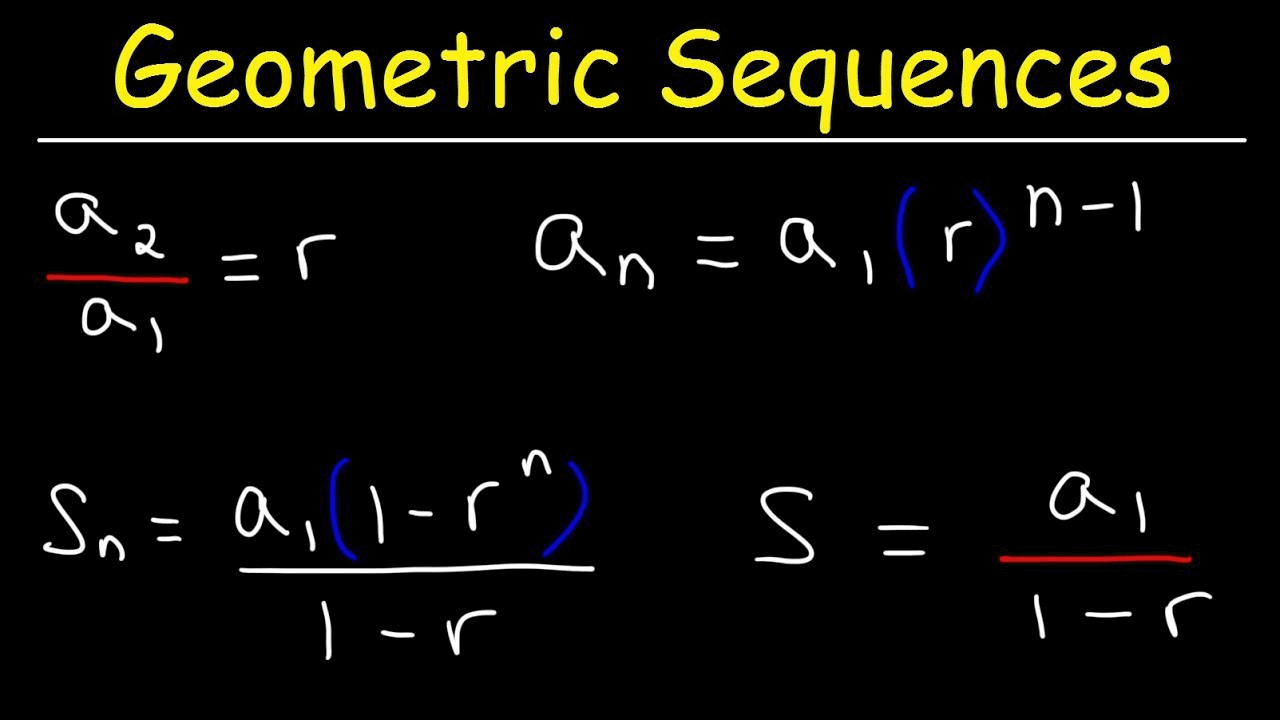Education

# How To Find The Value Of The Common Ratio In Geometric Series

Are you stuck in how you can calculate the value of the common ratio in geometric series?  Then you don’t need to stress yourselves out because we have got you a cover. But before going into more details, you need to understand what a Geometric Sequence is?

Any term divided by the previous term is a constant in this equation. The common ratio of the sequence is the name given to this constant. Divide any term in the series by the last term to find the common ratio. The sequence will be a1 is the beginning of a geometric sequence, and r is the common ratio.

{ a1, a1r,a1r2,a1r3}

Let’s understand it by the help of an example, 7,14,28,56. In this example, we are going to find out the common ratio, so concentrate!

We will divide a2 over a1, which must be equal to a3 over a2 and a4 over a3 and a5 over a4. But you don’t need to divide every single two terms by each other. You need to do it across the first two and see if it is consistent across the terms.

In the above example, a2 is 14 and a1 is 7, and we get 2 when we divide it. Now, we will double-check it. Use your mind. What do you get when we multiply 2 with 14? Yes, it’s 28, and when we multiply 28 with 2, the answer turns out to be 56.

## How to Use a Geometric Sequence Calculator?

To put what you’ve learned to good use, it’s time for you to put what you’ve learned to good use and compute some things! You may use the geometric sequence calculator by calculator-online.net to get the most important values of a finite geometric sequence.  The standard ratio, the first term, the final term, and the number of terms are included in these figures. You must enter three values without summation components to get the fourth element in a geometric series sum calculator.

# The Sum of Geometric Series

Why do many people have a query about how to find the sum of geometric series? So far, it has been discussed that the collection of numbers are known as geometric sequences or geometric progressions.

When you attempt to add the terms of a geometric sequence, you may get some fascinating outcomes. It’s as easy as determining the sum of a linear number series when they have a finite geometric progression with a restricted number of terms. The sum of this geometric series may even be calculated by hand. but you can also look up an online geometric sequence calculator for the geometric series calculation. However, by experimenting with the geometric series formula, they may get more efficacies. A mathematical symbol (summation) is used to sum up every phrase that follows it.

# Conclusion

Finally, the nth term and the sum of the first nth terms of the geometric sequence may be calculated using the geometric series calculator. It shows all the mathematics needed to calculate the sequence, sum of series, and common ratios. To do all of the computations in the geometric sequence calculator, the geometric sequence formula is used.